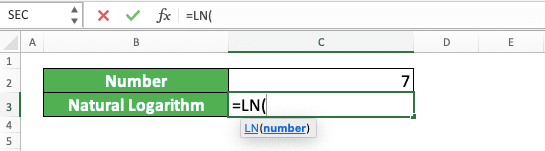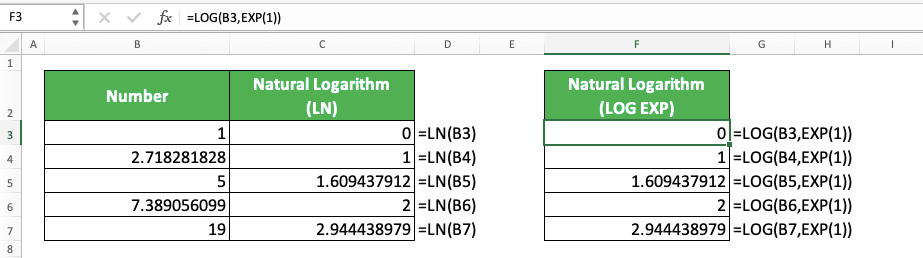How to Use the LN Function in Excel: Usabilities, Examples, and Writing Steps - Compute Expert

# How to Use the LN Function in Excel: Usabilities, Examples, and Writing Steps

Home >> Excel Tutorials from Compute Expert >> Excel Formulas List >> How to Use the LN Function in Excel: Usabilities, Examples, and Writing Steps

In this tutorial, you will learn how to use the LN function in excel completely.

When working in excel, we might sometimes need to find the natural logarithm of a number. A natural logarithm is a logarithm with the base of an e constant. Meanwhile, the e constant itself is Euler’s number, one of the most important mathematic constants, with a value of approximately 2.71828.

If we know how to use the LN function in excel, then we can easily calculate our number’s natural logarithm!

Want to know more about this LN function and how to use it properly in excel? Read this tutorial until its last part!

Disclaimer: This post may contain affiliate links from which we earn commission from qualifying purchases/actions at no additional cost for you. Learn more

## What is the LN Function in Excel?

LN is an excel function that can help you to get the natural logarithm of a number easily and fast.

## LN Usability

You can use LN to calculate the natural logarithm of your number in excel.

## LN Result

The LN result is a number that represents the natural logarithm of the number you input into it.

## Excel Version from Which We Can Start Using LN

We can start using LN in excel since excel 2003.

## The Way to Write It and Its Input

Here is the general writing form of LN in excel.

= LN ( number )

The only input you need to give in LN is the number you want to get the natural logarithm of. The number input itself must be a positive number.

## Example of Its Usage and Input

Here is an implementation example of LN in excel.As you can see in the example, the way to use LN in excel is quite easy. Just input the number we want to get the natural logarithm of and press Enter. By doing that, we will immediately get the result we want!

## Writing Steps

Need guidance when you write your LN formula in excel? Take a look at our LN writing steps below!

1. Type an equal sign ( = ) in the cell where you want to put the natural algorithm of your number2. Type LN (can be with large and small letters) and an open bracket sign after =3. Input the number you want to get the natural logarithm of4. Type a close bracket sign5. Press Enter
6. Done!## Check if Our Data isn’t a Logical Value: NOT ISLOGICAL

Your LN produces a wrong result or an error? Here are probably the reasons with the way to solve each of them.

• Reason: You input a negative number to LN
Solution: A negative number input will make your LN produce a #NUM error. Change your negative number to a positive one before you input it in your LN writing (the easiest way is probably by using the ABS formula)

• Reason: You input non-numeric data to LN
Solution: A non-numeric data input to LN will make it produces a #VALUE error. Make sure your LN input is a number and change it to a number if it isn’t

• Reason: You use LN to calculate the logarithm of your number
Solution: You use LN to calculate the natural logarithm of a number in excel, not its logarithm. To calculate the logarithm, you should use the LOG formula

## LN Alternative: LOG EXP

As LN calculates logarithm with the number e base, you can substitute it with the LOG and EXP combination.

LOG is a logarithm calculation function in excel while EXP is a function that lets you produce a powered e constant. By combining them, you can get the same result as LN in excel.

Here is the general writing form of the LOG and EXP combination that mirrors LN.

= LOG ( number , EXP ( 1 ) )

We use the input of 1 in EXP because we want our logarithm calculation base to be one e constant. Just like the logarithm base we use in LN.

Here is the implementation example of the two formulas combined, with its comparison to LN.As you can see, we get the same natural logarithm from LN and LOG EXP. That is because we use the same base for our logarithm here (one e constant).

You may want to use this alternative method should you need to calculate natural logarithm and other logarithm in one go.

## Exercise

After you have learned how to use LN in excel, now let’s do an exercise!

### Instructions

Use LN to answer all the questions in the appropriate gray-colored cell!
1. What is the natural logarithm of the number on the no. 1 row?
2. What is the natural logarithm of the number on the no. 2 row? Change the number in your input if necessary!
3. What is the natural logarithm of the number on the no. 3 row? Change the number in your input if necessary!

LN can process a number stored as text normally in excel, unlike some other excel calculation functions.

Related tutorials you should learn too:

Get updated excel info from Compute Expert by registering your email. It's free!